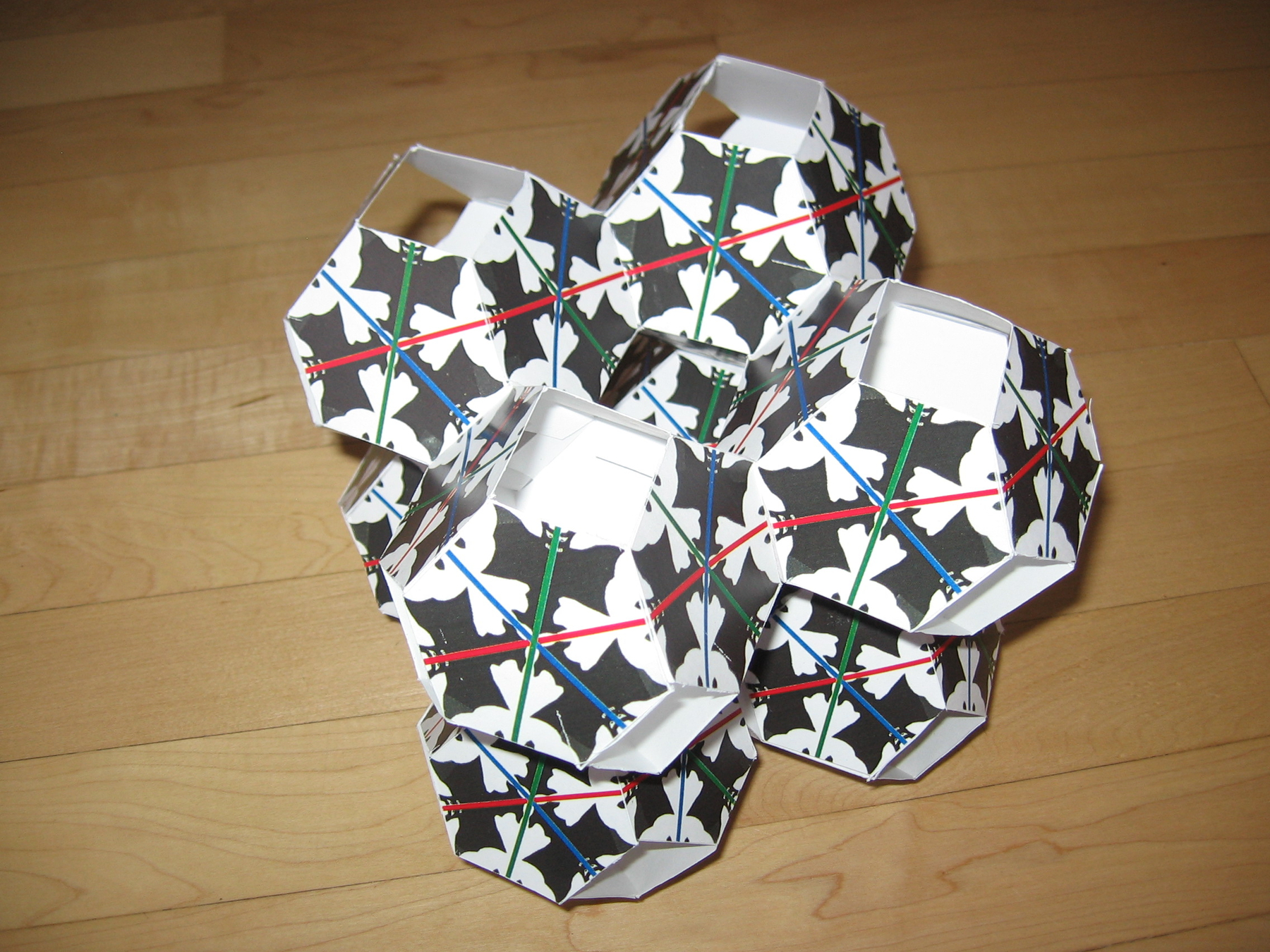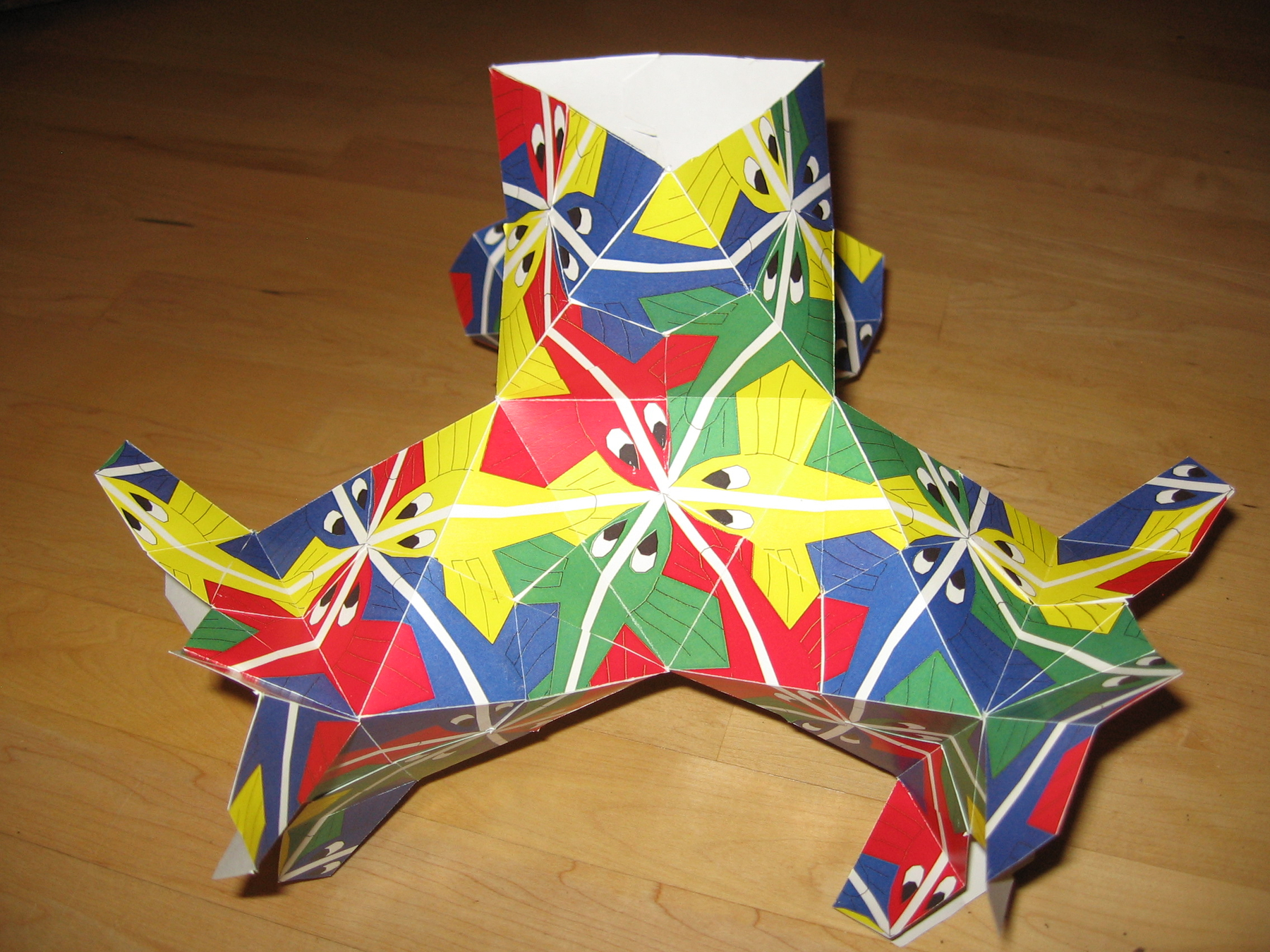# Doug Dunham

Professor of Computer Science
Department of Computer Science, University of Minnesota Duluth
Duluth, Minnesota, USA

The goal of my art is to create aesthetically pleasing repeating patterns related to hyperbolic geometry. In the past I have drawn patterns on the hyperbolic plane itself. But the pictures below are of patterns on triply periodic polyhedra in Euclidean 3-space, the first two of which are regular. The patterns are inspired by those of M.C. Escher, and like Escher's patterns, they have no gaps or overlaps. These polyhedra are related to the hyperbolic plane in two steps. First, the polyhedra are approximations to three triply periodic minimal surfaces (the vertices of the polyhedra all lie on the corresponding minimal surface). Second, since the minimal surfaces have negative curvature, the hyperbolic plane has the same large scale geometry as their universal covering surface.Angular Fish on the [6,6|3} Polyhedron
12x12x12 inches
Color printed cardboard
2012

This is a pattern of red, green, and blue angular fish on the regular triply periodic polyhedron composed of regular hexagons meeting six at each vertex, which is denoted by the modified Schläfli symbol {6,6|3} (the 3 indicates that there are 3-sided holes in it). The white backbones of the fish of any one color lie along (infinite) Euclidean lines that are embedded in the polyhedron. Three of these lines of fish, one of each color, pass through each vertex. The fish swim in one direction along the backbone lines, so the fish of one color enter a vertex from one hexagon and exit the vertex into the "opposite" hexagon. There are 3-fold color symmetries generated by 120-degree rotations about two kinds of axes of symmetry: those that go through the vertices and those that are perpendicular to the centers of the hexagons.Angels and Devils on the {6,4|4} Polyhedron
10x10x10 inches
Color printed cardboard
2012

This is a pattern of angels and devils (inspired by M.C. Escher) on the regular triply periodic polyhedron composed of regular hexagons meeting four at each vertex, which is denoted by the modified Schläfli symbol {6,4| 4} (the last 4 indicates that the polyhedron has square holes). The axes of bilateral symmetry of the angels and devils are colored red, green, and blue. Disregarding the pattern, the polyhedron has the same symmetries as the cubic lattice. The pattern of colored bilateral symmetry lines has 3-color symmetries about the 3-fold axes perpendicular to the centers of the hexagons. These are the 3-fold axes of the "body" diagonals of the corresponding cubic lattice.Fish on the {3,8} Polyhedron
20x20x20 inches
Color printed cardboard
2012

This is a pattern of fish (inspired by M.C. Escher's Circle Limit III) on the regular triply periodic polyhedron composed of equilateral triangles meeting 8 at each vertex, which can be denoted by the Schläfli symbol {3,8}. It is formed from octahedral hubs which have octahedral struts that connect the hubs; the struts are on alternate faces of the hubs. This polyhedron is an approximation to a minimal surface which is the boundary between two congruent, complementary solids, both in the shape of a "thickened" diamond lattice (the hubs are the carbon atoms and the struts are the atomic bonds). There are fish of four colors. The blue fish all swim around the "waists" of the struts. The yellow, green, and red fish swim along lines that approximate the Euclidean lines that are embedded in the minimal surface. In the image above the yellow fish swim right to left, the green fish swim from lower left to upper right, and the red fish swim from upper left to lower right.